## Unsections/Cones and “Tom vs Jerry” ambiguity

Unsections/Cones and “Tom vs Jerry” ambiguity:
why no single-valued ansatz is possible and Minkowski ambiguity is the thing to expect

[Miles Reid-like notation]
Consider two del Pezzo threefolds of degree 6.
Let Jerry be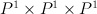$P^1 \times P^1 \times P^1$
and Tom be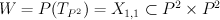$W = P(T_{P^2}) = X_{1,1} \subset P^2 \times P^2$ (hyperplane section of product of two planes in Segre embedding).
It is known that Tom and Jerry are not fibers of a flat family.

Tom has period sequence 6,
Jerry is grdb[520140 and has period sequence 21.

Their half-anticanonical section is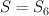$S = S_6$ (del Pezzo surface of degree 6).
So both Tom and Jerry can be degenerated to the same Gorenstein toric Fano threefold — anticanonical cone over S.

This cone has just one integral point except origin and vertices.
Let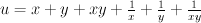$u = x + y + xy + \frac{1}{x} + \frac{1}{y} +\frac{1}{xy}$
be the normalized Laurent polynomial for the honeycomb (fan polytope of S).

Note that honeycomb has two different Minkowski decompositions — as sum of three intervals and as sum of two triangles.

These decompositions correspond to two different decompositions of (u+G) into the product of Laurent polynomials [for two different values of G (G=2 and G+3)]: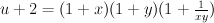$u+2 = (1 + x) (1 + y) (1 + \frac{1}{xy})$

and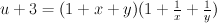$u+3 = (1 + x + y) (1 + \frac{1}{x} + \frac{1}{y})$

General Laurent polynomial for the cone over S
has the form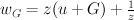$w_G = z (u + G) + \frac{1}{z}$

The most interesting thing is the following:

if we choose $G=2$ then w is mirror of Jerry,
but if we choose $G=3$ then w is mirror of Tom.

Moreover applying mutation we can transform w to terminal Gorenstein polynomials:

————
[tom]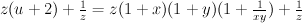$z (u+2) + \frac{1}{z} = z (1+x)(1+y)(1+\frac{1}{xy}) + \frac{1}{z}$

becomes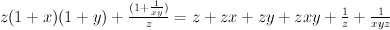$z (1+x)(1+y) + \frac{(1+\frac{1}{xy})}{z} = z + zx + zy + zxy + \frac{1}{z} + \frac{1}{xyz}$

by applying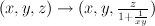$(x,y,z) \to (x,y,\frac{z}{1+\frac{1}{xy}})$.

This corresponds to STD of Tom.
It looks nicer after monomial transformation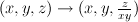$(x,y,z) \to (x,y,\frac{z}{xy})$: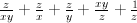$\frac{z}{xy} + \frac{z}{x} + \frac{z}{y} + \frac{xy}{z} + \frac{1}{z}$

————
[jerry]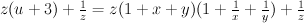$z (u+3) + \frac{1}{z} = z (1+x+y)(1+\frac{1}{x}+\frac{1}{y}) + \frac{1}{z}$

becomes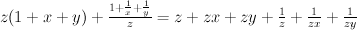$z (1+x+y) + \frac{1 + \frac{1}{x} + \frac{1}{y}}{z} = z + zx + zy + \frac{1}{z} + \frac{1}{zx} + \frac{1}{zy}$

by applying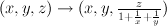$(x,y,z) \to (x,y,\frac{z}{1+\frac{1}{x}+\frac{1}{y}})$

This is simply Laurent polynomial for the smooth model of Jerry: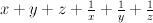$x+y+z+\frac{1}{x} + \frac{1}{y} + \frac{1}{z}$
after monomial transformation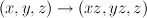$(x,y,z) \to (xz,yz,z)$.

————-

So Laurent phenomenon distinguishes degenerations of different varieties to the same singular and does not mix them.

### One Comment

1.Sergey says:

Tom, Jerry and Spike.

If in the previous scenario we look for bigger brothers of Tom and Jerry with index 1,
then we have a pleasant surprise – not only we get 3.1 and 2.6, but also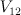$V_{12}$.

For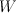$W$ and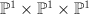$\PP^1 \times \PP^1 \times \PP^1$ we constructed mirrors
as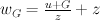$w_G = \frac{u+G}{z} + z$ where$u = x+y+xy + \frac{1}{x} + \frac{1}{y} + \frac{1}{xy}$,
and G is either 2 or 3.

Regularized I-series for 2.6 and 3.1 has the same coefficients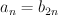$a_n = b_{2n}$,
so their mirrors should be related to squares of mirrors for$W$ and$\PP^1 \times \PP^1 \times \PP^1$.

Consider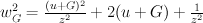$w_G^2 = \frac{(u+G)^2}{z^2} + 2(u+G) + \frac{1}{z^2}$.
Obviously it has the same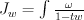$J_w = \int \frac{\omega}{1-tw}$ as its isogeneous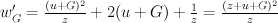$w'_G = \frac{(u+G)^2}{z} + 2(u+G) + \frac{1}{z} = \frac{(z+u+G)^2}{z}$

Newton polytope for this kind of Laurent polynomials have number 432464 in grdb.

We can check that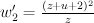$w'_2 = \frac{(z+u+2)^2}{z}$ has period sequence 11 and is mirror to 2.6,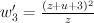$w'_3 = \frac{(z+u+3)^2}{z}$ has period sequence 22 and is mirror to 3.1.

But we can have even more – I find it very amusing that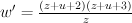$w' = \frac{(z+u+2)(z+u+3)}{z}$ has period sequence 7 and is mirror to$V_{12}$.

Since singular toric variety in question is again a cone over surface, probably it won’t be hard to prove the existence of degenerations.